CAT  >  CAT Quant Mock Test - 2

# CAT Quant Mock Test - 2 - CAT

Test Description

## 25 Questions MCQ Test - CAT Quant Mock Test - 2

CAT Quant Mock Test - 2 for CAT 2023 is part of CAT preparation. The CAT Quant Mock Test - 2 questions and answers have been prepared according to the CAT exam syllabus.The CAT Quant Mock Test - 2 MCQs are made for CAT 2023 Exam. Find important definitions, questions, notes, meanings, examples, exercises, MCQs and online tests for CAT Quant Mock Test - 2 below.
Solutions of CAT Quant Mock Test - 2 questions in English are available as part of our course for CAT & CAT Quant Mock Test - 2 solutions in Hindi for CAT course. Download more important topics, notes, lectures and mock test series for CAT Exam by signing up for free. Attempt CAT Quant Mock Test - 2 | 25 questions in 35 minutes | Mock test for CAT preparation | Free important questions MCQ to study for CAT Exam | Download free PDF with solutions
 1 Crore+ students have signed up on EduRev. Have you?
CAT Quant Mock Test - 2 - Question 1

### DIRECTIONS for question: Select the correct alternative from the given choices. Find the ratio in which the line joining the points (-3, 3) and (2, 6) is divided by they-axis.

Detailed Solution for CAT Quant Mock Test - 2 - Question 1
The ratio in which the y-axis divides the line joining (x1, y1) and (x2, y2) is – x1, : X2 = - (- 3): 2 = 3 : 2

CAT Quant Mock Test - 2 - Question 2

### DIRECTIONS for questions: Select the correct alternative from the given choices. There are 50 students divided into two groups, P and Q, with 20 and 30 students respectively. One student from group P shifts to group Q. If as a result of this, the average weight of group P decreases, while the average weight of group Q increases, which of the following holds true?

Detailed Solution for CAT Quant Mock Test - 2 - Question 2

Let the average weights of students in groups P and Q be p and q respectively. Total weights of students in groups P and Q are 20p and 30q respectively. Let the weight of the student who shifted from group P to group Q be w. After the shifting of the student,

the average weights of groups P and Q become 20p – w / 19 and 30q + w / 31respectively.

Given that20p – w / 19< p. Hence w > p.

Also given that 30q + w / 31> q. Hence w > q.

Alternative Solution

If a higher-average group joins or a lower-average group leaves, the average increases.

If a lower-average group joins or a higher-average group leaves, the average decreases.

The student leaves P and the average decreases. Therefore the student’s weight is more than initial (as well as the final) average weight of P. He joins Q and the average increases. Therefore, the student’s weight is more than the initial (as well as final) average weight of Q.

CAT Quant Mock Test - 2 - Question 3

### DIRECTIONS for questions: Select the correct alternative from the given choices. What is the least distance from the point (4, 0) to the curvex2 + y2 − 6y − 12x + 28 = 0?

Detailed Solution for CAT Quant Mock Test - 2 - Question 3
x2 + y2 - 6y - 12x + 28 = 0

x2 - 12x + 36 + y2 - 6y + 9 + 28 = 36 + 9

(x - 6)2 + (y - 3)2 = 17

The above curve is a circle with centre at (6, 3) and radius √17 units. The least distance between the point and circle

= ∣Distance between the point and centre - Radius of the circle∣

Distance between the point (4, 0) and the center (6, 3) is√(6 – 4)2 + (3 – 0)2

= √22 + 32 = √13

=> least distance =√17 - √13 units.

CAT Quant Mock Test - 2 - Question 4

DIRECTIONS for questions: Select the correct alternative from the given choices.

During the placements in an MBA college, students were placed in four different sectors -Marketing (M), Finance (F), Operations (O) and IT (I). The averages of the annual CTCs of the students placed in M, F, O together and F, O, I together are Rs.13 lakh and Rs.14 lakh respectively, while the averages of the annual CTCs of the students placed in M, O, I together and M, F, I together are Rs.12 lakh and Rs.15 lakh respectively. Which of the following (in Rs. lakhs) could possibly be the average annual CTC of all the students placed?

I. 12.5

II. 13.6

III. 14

Detailed Solution for CAT Quant Mock Test - 2 - Question 4
Let the number of students who get placed in Finance, Marketing, Operations and IT be f, m, o and i.

The average of the annual CTC’s of the students who are placed in M, F and O is 13.

=> The sum of the annual CTC’s of the students who are placed in M, F, O is 13(m + f + o) → (1)

Similarly, the sum of the annual CTC's of the students who are placed in F, O, I is 14(f + o + i) → (2)

The sum of the annual CTC’s of the students who are placed in M, O, I is 12(m + o + i) → (3)

The sum of the annual CTC’s of the students who are placed in M, F, I is 15(m + f + i) → (4}

We can observe that each of the four different sectors appears in exactly three of (1), (2), (3) and (4).

∴The average annual CTC of the students placed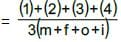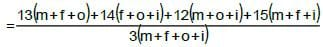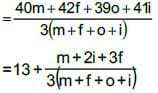Since, m + 2i + 3f will be less than 3 ( m + f + o + i), then average annual CTC of the students will be between 13 and 14.

∴Among the given options only II is possible.

CAT Quant Mock Test - 2 - Question 5

DIRECTIONS for questions: Select the correct alternative from the given choices.

A function f(x) is defined for a non-zero variable x, such that the value of f(y), where y = x + 1 / x, equals x2 + 1 / x2equals . The function f(x) can be

Detailed Solution for CAT Quant Mock Test - 2 - Question 5

Given that for a variable ‘x’, ‘f’ is such that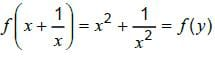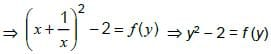Hence the function should be f(x) = x2 - 2

CAT Quant Mock Test - 2 - Question 6

DIRECTIONS for questions: Select the correct alternative from the given choices.

Five identical tennis balls are kept in a cylindrical container whose length is such that the five balls just fit into it in a single column. Find the ratio of the volume of the unoccupied portion of the container to that of the occupied portion, given that each ball is of the maximum possible size that can fit inside the container.

Detailed Solution for CAT Quant Mock Test - 2 - Question 6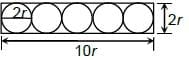The above figure gives the side view of the container and the balls.

Length of the box = 5 x 2r = 10r

Radius of the box = r

Volume of the box = πr2 x 10r = π10r3

Total volume of the five balls = 5 x 4/3 x π x r3 = 20r3π / 3

Unoccupied volume = 10πr3(1 – 2/3) = 10πr3(1 /3).

∴Required ratio = 10πr3: 20/3 πr3 =1:2

CAT Quant Mock Test - 2 - Question 7

DIRECTIONS for questions: Select the correct alternative from the given choices.

The arithmetic mean of a set of numbers was calculated as 24. However, the frequency of one of the numbers was erroneously taken as 6, instead of 9. As a result, the arithmetic mean calculated was 5 less than the actual mean. If all the numbers in the set are integers, then which of the following could be the sum of all frequencies?

Detailed Solution for CAT Quant Mock Test - 2 - Question 7
Let the number whose frequency is taken erroneously be x.

Let the sum of frequencies initially considered be k

=> The actual sum of frequencies = k + 3

Calculated mean = 24

=> calculated sum of all observations = 24k

Actual sum = 24k + 3x

Actual mean = 24k + 3x / k + 3 = 24 + 5 = 29

=> 24k + 3x = 29k + 87

=> 5k = 3x - 87

=> k = 3(x – 29) / 5

As both k and x are integers, x - 29 must be a multiple of 5 and k must be a multiple of 3.

=> k + 3 must be a multiple of 3.

Among the given options, only 42 is a multiple of 3.

CAT Quant Mock Test - 2 - Question 8

DIRECTIONS for questions : Select the correct alternative from the given choices.

Find the sum of the first n terms of the series: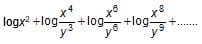Detailed Solution for CAT Quant Mock Test - 2 - Question 8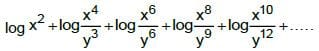2 logx + 4 logx - 3 logy + 6 logx - 6 logy + 8 logx - 9 logy + + 2n logx - 3(n - 1) logy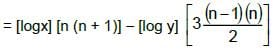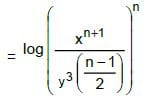Alternative Solution

This question can also be answered by eliminating the answer choices. By substituting n = 1, choices (b), (c) get eliminated and for n = 2; choice (d) is eliminated.

∴Only choice (a) can be the answer.

CAT Quant Mock Test - 2 - Question 9

DIRECTIONS for question: Select the correct alternative from the given choices.

WIMCO employs a total of 75 units to make coir products for export. The total monthly revenue from all the units is Rs.10,00,000. The total manufacturing cost and the total overheads (both of which vary with the number of units working) for all the units are Rs.1,50,000 and 1,00,000 every month respectively. If, in one month, the total profit reduced by Rs.35,000, how many units, on an average, were not working everyday during that month?

Detailed Solution for CAT Quant Mock Test - 2 - Question 9
Total revenue = 10,00,000

Manufacturing Expenses = 1,50,000

Total Expenses = 2,50,000

Profit = 7,50,000

Profit/Unit = 10,000

Reduction in profit = 35,000 35,000

∴Average number of idle units = 35,000 / 10,000 =3.5

CAT Quant Mock Test - 2 - Question 10

DIRECTIONS for questions: Select the correct alternative from the given choices.

The breadth of a rectangle is decreased by 25%, as a result of which the perimeter of the rectangle decreased by 10%. By what percentage should the length of the rectangle now be increased, such that the perimeter of the rectangle will be the same as the initial one (i.e., before the breadth was decreased)?

Detailed Solution for CAT Quant Mock Test - 2 - Question 10
Given, Let the length and breadth be ℓ and b respectively.

2(ℓ + 0.75b) = 0.9 x (2(ℓ + b))

=> ℓ + 0.75b = 0.9ℓ + 0.9b

=>0.1 ℓ = 0.15b

=>ℓ : b = 3 :2

Let ℓ = 30 and b = 20

=> Present ℓ, b = (30, 15), i.e., after decrease in breadth.

Now, the length should be increased by 5 in order to compensate (i.e., retain ℓ + b = 30 + 20 = 50) for the decrease in breadth.

Hence 5 / 30 x 100 = 16.67%

CAT Quant Mock Test - 2 - Question 11

DIRECTIONS for questions: Select the correct alternative from the given choices.

What is the radius (in cm) of the largest possible circle that can be cut out from a quadrant of a circle of radius 6 cm?

Detailed Solution for CAT Quant Mock Test - 2 - Question 11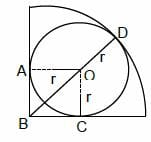The largest circle that can be cut from the quadrant must touch the two radii and the arc of the quadrant. Let its radius be r.

AOCB is a square where O is the centre of the circle to be cut.

= r√2 + r = 6

=> r = 6 / √2 + 1 = 6(√2 – 1)

Alternately, the radius of the smaller circle must be less than half the radius of the larger circle. Hence r < 3.="" by="" simple="" calculation,="" only="" choice="" (c)="" />

CAT Quant Mock Test - 2 - Question 12

DIRECTIONS for questions: Select the correct alternative from the given choices.

Find the positive square root of 13 + √48 + √72 + √96.

Detailed Solution for CAT Quant Mock Test - 2 - Question 12
Let the required square root be √a + √b + √c

Now, (√a +√b +√c =a+b+c+2√ab +2√bc +2√ac

13+√48 + √72+ √96 = 13+2√12 + 2√18 + 2√24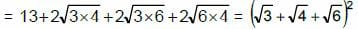Therefore the square root is

√a +√b +√c =√4+√3+√6

i.e. 2 + √3(√2+ 1)

Alternative Solution

Choices (A) and (C) can be easily eliminated since none of the surds in the given expression has √5 as factor. Choice (B) is also eliminated since √2x√8 would make the rational part much more (2 + 8 + 3 + 4= 17) than 13 (in the given expression). Hence, by elimination, Choice (d).

CAT Quant Mock Test - 2 - Question 13

DIRECTIONS for question: The question is followed by two statements, I and II. You have to decide whether the information provided in the statements is sufficient for answering the question and select the correct answer option.

Gayatri spent less than Rs.180 to buy three kg of each of rice, wheat and jowar. Which of the three cereals she bought was the cheapest?

I. 4 kg rice and 2 kg wheat cost less than 2 kg rice and 4 kg wheat.

II. 2 kg wheat and 4 kg jowar cost the same as 4 kg rice and 2 kg jowar.

Detailed Solution for CAT Quant Mock Test - 2 - Question 13
Let the cost of 1 kg of rice, 1 kg of wheat and 1 kg of jowar be₹r, ₹w, ₹j respectively.

From I alone, we have

4r + 2w< 2r+4w

=> r < w.

It is not sufficient.

From n alone we have

2w + 4j = 4r + 2j => w+j= 2r

It is not sufficient

By combining both I and II, we can say that w> r> j.

CAT Quant Mock Test - 2 - Question 14

DIRECTIONS for questions: Select the correct alternative from the given choices. Robert bought an equal number of each of three kinds of articles, whose cost prices were Rs.8, Rs.25 and Rs.38 per article respectively. If, on the whole, he spent Rs.2,769, what is the total number of articles that he bought?

Detailed Solution for CAT Quant Mock Test - 2 - Question 14
Price of each type of article is ₹8, ₹25, ₹38.

Let us assume Robert bought x articles of each kind.

Total Amount spent = 8x + 25x + 38x = 2769

=> 71x = 2769

=> x = 39

∴Robert bought 39 articles of each kind.

=>A total of 117 articles.

CAT Quant Mock Test - 2 - Question 15

DIRECTIONS for questions: Select the correct alternative from the given choices.

The range of cos6x + sin6x is

Detailed Solution for CAT Quant Mock Test - 2 - Question 15
Let E = cos6x + sin6x

= (cos2x + sin2x)3 - 3 cos2x sin2x (cos2x + sin2x)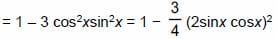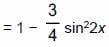For all x, 0 ≤ sin2 2x ≤ 1.

If sin2 2x = 0, then E = 1 and if sin2 2x= I, then E = 1 / 4

∴The required range is [1 / 4, 1]

Alternative Solution

Since sin6x + cos6x is always positive, choices (A) and (D) can be eliminated. Further, since both sinx and cosx cannot be simultaneously zero, even choice (C) is eliminated. Hence, choice (b).

CAT Quant Mock Test - 2 - Question 16

DIRECTIONS for questions: Select the correct alternative from the given choices.

A milkman has two containers, containing mixtures of milk and water. The first container has 110 litres of a mixture of milk and water in the ratio of 5 : 6. The second container has a mixture of milk and water in the ratio of 7 : 3. How much mixture should be taken from the second container and added to the first so that the ratio of the milk and water in the first container will become 6 : 5?

Detailed Solution for CAT Quant Mock Test - 2 - Question 16
The quantity of milk in the first container = 50 litres

Quantity of water in the first container = 60 litre.

Let the quantity of mixture added from the second container be 10x. 50 + 7x : 60 + 3x = 6:5

=>x= 6.471

Hence, 10x= 64.71 litres of mixture must be added.

CAT Quant Mock Test - 2 - Question 17

DIRECTIONS for questions: Select the correct alternative from the given choices.

A man has a special rechargeable electric vehicle, which can maintain a speed ofx km/hr for (100 − x) minutes, after which it has to be recharged. What is the maximum distance (in km) that the man can cover on the vehicle without recharging in between?

Detailed Solution for CAT Quant Mock Test - 2 - Question 17

If the speed of x km/hr be maintained for 100 - x minutes, the maximum distance that 50 can be covered occurs when x = 100 -x, i.e., x - 50 and it is 50 / 60 (50) = 41 x 2 / 3km

CAT Quant Mock Test - 2 - Question 18

DIRECTIONS for question: Select the correct alternative from the given choices.

Ashok told his son, “x years ago, my age was twice your age and 5x years ago, my age was thrice your age.” If the difference of their present ages is 24 years, find the sum of their present ages (in years).

Detailed Solution for CAT Quant Mock Test - 2 - Question 18
Let the present ages of Ashok and his son be A years and S years respectively.

Given A - S = 24

=> A = S + 24 — (1)

Also, x years ago (A- x) = 3(S - x)

=> (S + 24 - x) = 2(S - x)— (2)

Similarly, 5x years ago

(S + 24 - 5x) = 3(S - 5x) — (3)

Solving (2) and (3), x = 3, S = 27 and A = 51=> S + A = 78

Alternative solution

Since the difference A - S is even (i.e., 24) the sum also must be even. We could check for choice (B) and (D). Hence choices (1) and (3) are eliminated.

Further, for choice (B)

A + S = 78 and A - S = 24 => A = 51, S = 27

By some observation. (51 - 3) = 2(27 - 3) and (51 - 15)

= 3(27 - 15) i.e., x = 3

CAT Quant Mock Test - 2 - Question 19

At a parking lot, there are total of 50 two-wheelers and four-wheelers. The total number of wheels of these vehicles is 160. Find the number of two-wheelers at the parking lot.

Detailed Solution for CAT Quant Mock Test - 2 - Question 19

Let the numbers of two-wheelers and four-wheelers at the parking lot be T and F respectively

T + F = 50 and 2T + 4F = 160.

Solving these, T = 20 and F = 30.

CAT Quant Mock Test - 2 - Question 20

Find the length (in m) of the platform which a 600 m long train, travelling at 72 kmph, can cross in 50 seconds.

Detailed Solution for CAT Quant Mock Test - 2 - Question 20

The total distance travelled by train in 50 sec.

= 50 x 72 x 5 / 18 = 1000 m.

∴ The length of the platform = 1000 - 600 = 400 m

CAT Quant Mock Test - 2 - Question 21

A merchant purchased a pack of 15 bottles of soft drink, at a cost price of Rs.15 per bottle, and sold the pack at a profit of 20%. What is the additional profit (in Rs.) he would have made, if he had sold the pack at 15% more than what he sold it for?

[Enter your answer as a decimal value, rounded off to two decimal places.]

Detailed Solution for CAT Quant Mock Test - 2 - Question 21
The total cost of buying the pack = 225

He sold it at 225 x 1.2 = 270

Profit he made = 270 - 225 = 45

If he sold it the next day, the selling price increased by 15% (the increase in C.P. would not affect the merchant since he has already purchased the pack before the price change)

Next dayS.P. = 270 x 1.15 = 310.5

Profit if he sold it the next day = 310.5 - 225 = 85.5

Additional profit = 85.5 - 45 = 40.5

CAT Quant Mock Test - 2 - Question 22

A work can be done by P in 48 days, whereas Q and R together can do the same work in 20 days. If Q is thrice as efficient as R, then P and R can do the work in

Detailed Solution for CAT Quant Mock Test - 2 - Question 22
As Q is thrice as efficient as R, when they work together, they work with 4 times the efficiency of R. If only R works, he will take 4(20) = 80 days to complete the work.

∴ Time taken by P and R to complete the same work is (80)(48) / 80 + 48 = 30 days.

CAT Quant Mock Test - 2 - Question 23

This year, during the months of January and February, each day Ramu went to a fruit shop and bought exactly three varieties of fruits, such that for any two days, he bought at least one variety of fruit on one of the days that was different from what he bought on the other day. What is the minimum number of different varieties of fruits that he could have bought during that period?

Detailed Solution for CAT Quant Mock Test - 2 - Question 23
Let the minimum number of varieties be n. Now, we have to select 3 fruits from these n. We have to choose n such that the number of selections (i.e., combinations of three out of n fruits) should be greater than (or) equal to the number of days, i.e., 31 + 28 = 59. i.e., nC3 ≥ 59

for n = 8 (8)(7)(6) / 6 = 56 < 59

So minimum possible n is 9.

CAT Quant Mock Test - 2 - Question 24

The following graph plots the time of travel (in seconds) and the speed of travel (in meters per second) of a particle. What is the total distance (in m) travelled by the particle from t = 0 to t = 4 seconds?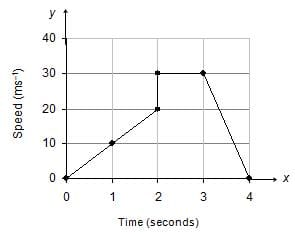Detailed Solution for CAT Quant Mock Test - 2 - Question 24
The x-axis has time in secs and the y-axis has speed in mts. Hence the area of the plotted region will give us the distance travelled in m (not km). Area of the graph is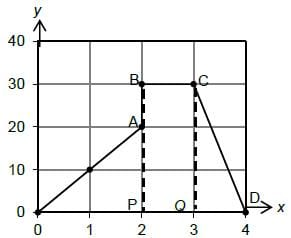= Area of AOAP + Area of rectangle PBCQ + Area of triangle QCD

= (1 / 2 x 2 x 20) + (1 x 30) + (1 / 2 x 1 x 30) = 20 + 30 + 15 = 65 m

∴ Distance travelled is 65 m

CAT Quant Mock Test - 2 - Question 25

If the area enclosed by the lines 3x − 2y + 6 = 0, 3x + 5y − 15 = 0 and the x-axis is A sq. units, find the value of 4A.

Detailed Solution for CAT Quant Mock Test - 2 - Question 25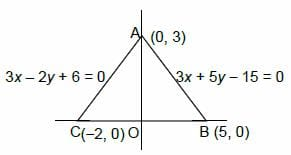The given lines 3x - 2y + 6 = 0 and 3x + 5y - 15 = 0 intersect on the y-axis at (0, 3). The area of the triangle formed by the lines 3x - 2y + 6 = 0, 3x + 5y - 15 = 0 and x-axis will be 1 / 2 x AO x BC = 1 / 2 x 3 x 7 = 10.5 sq.units. A = 10.5

∴4A =42

Information about CAT Quant Mock Test - 2 Page
In this test you can find the Exam questions for CAT Quant Mock Test - 2 solved & explained in the simplest way possible. Besides giving Questions and answers for CAT Quant Mock Test - 2, EduRev gives you an ample number of Online tests for practice

### How to Prepare for CAT

Read our guide to prepare for CAT which is created by Toppers & the best Teachers(Scan QR code)